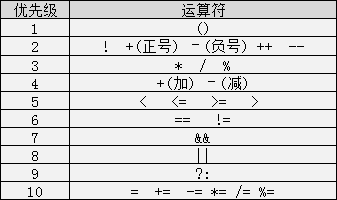signed

QiShunwang

“诚信为本、客户至上”

# 8.运算符号

2021/3/21 8:27:12   来源：

# 8.运算符号

※ 算术运算符

※ 赋值运算符

※ 关系运算符

※ 逻辑运算符

※ 三目运算符

``````加法：+

``````

``````9.0/3=3.000000
9/3=3
9/2=4
``````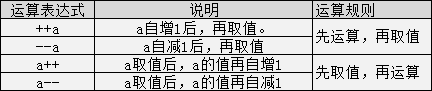``````int a=100;
a++:100,101
++a:101,102
a--:100,99
--a:99,98
//区别就是是先取值还是先运算
``````

``````int a = 3;
a += 5; //变相等于a=a+5
``````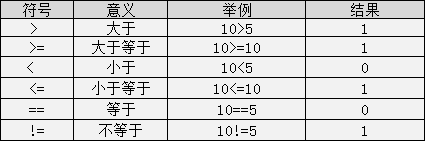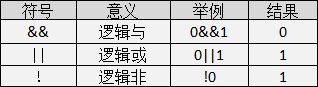C语言中的三目运算符：“?:”其格式为：

``````表达式1？表达式2：表达式3;
``````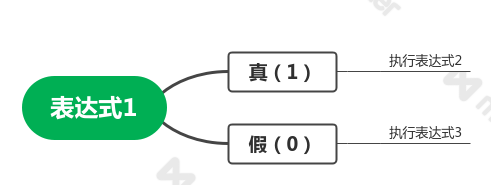``````#include <stdio.h>
int main()
{
//定义兜里的钱
double money = 12;
//定义打车回家的费用
double cost =  11.5;
printf("小编能不能打车回家呢：");
//输出y小编就打车回家了，输出n小编就不能打车回家
printf("%c\n",money>=cost?'y':'n');
return 0;
}
``````